Next: Rectangular Coordinates, Strip and Up: Rectangular Coordinates. Finite Bodies, Previous: Parallelepiped, steady GF, double-

### Pseudo GF. Rectangular coordinate, type 2 boundaries.

When all boundaries are type 2, the usual differential equation for G cannot be satisfied. However, a pseudo Green's function, denoted GPS, can be used that satisfies (cases X22, X22Y22, and X22Y22Z22):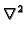GPS = -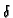(r - r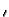) +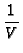(15)

with homogeneous boundary conditions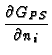= 0,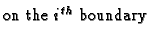(16)

Here(r) represents the appropriate Dirac delta function for each body shape and V is the appropriate volume (L for the slab, LW for the rectangle, and LWH for the parallelepiped). The physical reason for the pseudo GF is that when all of the boundaries are insulated, there is no heat sink'' to which heat, introduced by the infinitessimal heat source, can flow. The additional term in the differential equation balances the heat source. The pseudo GF takes on the same forms as the usual GF, and most of the previous discussion applies to the pseudo GF. To find the temperature, the pseudo GF must be applied in such a way that the heating terms satisfy an energy balance; the heat introduced by internal heating and the heat flowing through the boundaries must sum to zero. Also, the average temperature in body appears as an additive constant. The integral equation for the temperature is given by

 T(r) =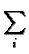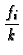GPS(r, ri)dsi+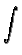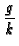GPS(r, r)dv+ < T > (17)

The sum is over all faces of the body and < T > is the spatial average temperature in the body.Next: Rectangular Coordinates, Strip and Up: Rectangular Coordinates. Finite Bodies, Previous: Parallelepiped, steady GF, double-
Kevin D. Cole
2002-12-31